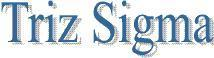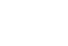Basic Statistics Mean, Median , And Mode The mean, for population and for a sample, is a measure of central tendency or typicality, i.e. where is the process centered, where most of the units are. The median and mode are used for circumstances where the data is skewed or associated with counts. An example of where median would be appropriate would be pay or compensation. To state an average or mean would overstate the situation. In 1987 for instance, the median population annual income worldwide was \$50 U.S. Yet the overall global average would have been in the thousands of dollars. Thus, if a case were being made that there is a poverty problem, then using the median would have more impact. The mode is often used in polling or survey situations where we would like to see what is the most common answer to a given question MEASURES OF DISPERSION : There are a number of measures to quantitatively relay dispersion  variance, standard deviation and range. The most commonly used measure is standard deviation. Put in its simplest terms, standard deviation is the average amount of variation we can expect across observations. Standard deviation is the average amount by which we expect a given occurrence to be away from the typical occurrence (i.e. the mean). Thus, if ones heart rate were at 70 and the average variation from observation to observation was 5 beats, the standard deviation would be 5. An important concept for measurement is the normal distribution and its link to standard deviation. The normal distribution is used to predict the number of observations that will be at a certain level of a process along with the ability to predict the number of observations above or below a given level of the process. The total area under the normal curve is 100% of all the observations or possibilities.The number of standard deviations associated with the proportion of observations is summarized below and shown in Figure : + & -1 standard deviation = 68.3 % of observations + & -2 standard deviations = 95.5% of observations + & - 3 standard deviations = 99.73% of observations